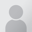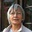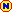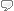# Flex polynomial regression

## French translation: régression polynomiale flexible

 16:36 Jul 11, 2017
English to French translations [PRO]
Bus/Financial - Mathematics & Statistics
 English term or phrase: Flex polynomial regression Bonjour, Comment traduiriez-vous "flex polynomial regression" dans le contexte suivant ? Légende d'un graphique : "95 percent confidence interval using flex polynomial regression". Merci d'avance :)Local time: 15:33
 French translation:régression polynomiale flexible Explanation:flex = flexible Polynomial regression extends the linear model by adding extra predictors, obtained by raising each of the original predictors to a power. Cubic regression uses three variables X, X2, and X3 as predictors. This is a simple way to provide a non-linear fit to the data. •Step functions cut the range of a variable into K distinct regions in order to produce a qualitative variable. This has the effect of fitting a piece wise constant function. •Regression splines are more flexible than polynomials and step functions, and are actually an extension of the two. The divide the range of X into K distinct regions. For each region, a polynomial function is fit to the data, however, the polynomials are constrained so that they join smoothly at the region boundaries or knots. These can provide and extremely flexible fit.
Selected response from:

Francois Boye
United States
Local time: 09:33
 Merci :)4 KudoZ points were awarded for this answer

4régression polynomiale flexible
 Francois BoyeAnne BohyDiscussion entries: 5

1 hr   confidence:flex polynomial regression
régression polynomiale flexible

Explanation:
flex = flexible

Polynomial regression extends the linear model by adding extra predictors, obtained by raising each of the original predictors to a power. Cubic regression uses three variables X, X2, and X3 as predictors. This is a simple way to provide a non-linear fit to the data.

•Step functions cut the range of a variable into K distinct regions in order to produce a qualitative variable. This has the effect of fitting a piece wise constant function.

•Regression splines are more flexible than polynomials and step functions, and are actually an extension of the two. The divide the range of X into K distinct regions. For each region, a polynomial function is fit to the data, however, the polynomials are constrained so that they join smoothly at the region boundaries or knots. These can provide and extremely flexible fit.

 Francois BoyeUnited StatesLocal time: 09:33Specializes in fieldNative speaker of:FrenchPRO pts in category: 16Merci :)Peer comments on this answer (and responses from the answerer)
neutral  Diego Delfino: This is what I thought as well (as posted in the discussione entry) did you find any result anywhere? I meant, french results, since I hadn't found any ghits.
 1 hr
-> Regression splines are more flexible than polynomials and step functions, and are actually an extension of the two.

16 hrs   confidence:flex polynomial regression

Explanation:
C'est probablement ceci (voir lien ci-dessous), mais seul le client pourrait le confirmer

Reference: http://fr.wikipedia.org/wiki/R%C3%A9gression_multivari%C3%A9...
 Anne BohyFranceLocal time: 15:33Works in fieldNative speaker of:FrenchPRO pts in category: 22Login or register (free and only takes a few minutes) to participate in this question.

You will also have access to many other tools and opportunities designed for those who have language-related jobs (or are passionate about them). Participation is free and the site has a strict confidentiality policy.

## KudoZ™ translation help

The KudoZ network provides a framework for translators and others to assist each other with translations or explanations of terms and short phrases.